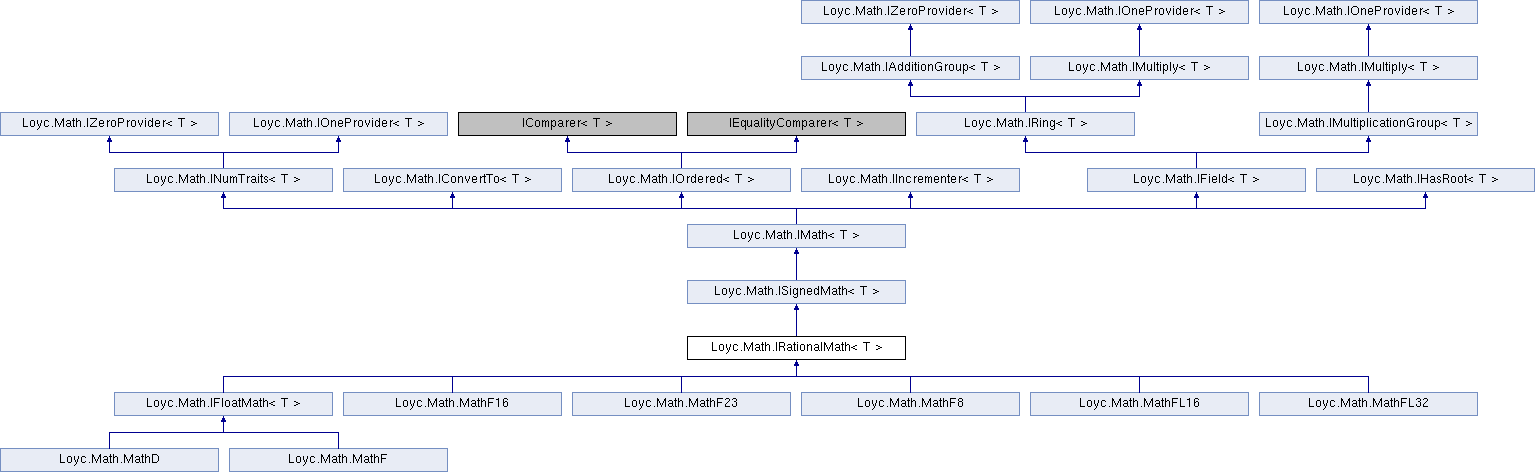Enhanced C# Language of your choice: library documentation

# Documentation moved to ecsharp.net

GitHub doesn't support HTTP redirects, so you'll be redirected in 3 seconds.

Loyc.Math.IRationalMath< T > Interface Template Reference

Use this interface for floating-point, fixed-point, and rational types. Rational types support reciprocal and negation. More...

Source file:
Inheritance diagram for Loyc.Math.IRationalMath< T >:## Remarks

Use this interface for floating-point, fixed-point, and rational types. Rational types support reciprocal and negation.

## Public Member Functions

Reciprocal (T a)Public Member Functions inherited from Loyc.Math.ISignedMath< T >
Negate (T a)Public Member Functions inherited from Loyc.Math.INumTraits< T >
bool IsInfinity (T value)
Returns true if the given value is infinite. More...

bool IsNaN (T value)
Returns true if the given value is not a number (can only be true for floats). More...

Floor (T value)
Gets the closest integer equal to or lower than the specified number. More...

Ceiling (T value)
Gets the closest integer equal to or higher than the specified number. More...Public Member Functions inherited from Loyc.Math.IConvertTo< T >
From (uint t)

From (int t)

From (ulong t)

From (long t)

From (double t)

Clip (uint t)

Clip (int t)

Clip (ulong t)

Clip (long t)

Clip (double t)Public Member Functions inherited from Loyc.Math.IOrdered< T >
bool IsLess (T a, T b)

bool IsLessOrEqual (T a, T b)

Abs (T a)

Max (T a, T b)

Min (T a, T b)Public Member Functions inherited from Loyc.Math.IIncrementer< T >
Returns a + 1. More...

SubOne (T a)
Returns a - 1. More...

NextHigher (T a)
Returns the next representable number higher than a. More...

NextLower (T a)
Returns the next representable number lower than a. More...Public Member Functions inherited from Loyc.Math.IAdditionGroup< T >

Add (T a, T b, T c)

Sub (T a, T b)Public Member Functions inherited from Loyc.Math.IMultiply< T >
Mul (T a, T b)Public Member Functions inherited from Loyc.Math.IMultiplicationGroup< T >
Div (T a, T b)

Shl (T a, int amount)

Shr (T a, int amount)

MulDiv (T a, T mulBy, T divBy)Public Member Functions inherited from Loyc.Math.IHasRoot< T >
Sqrt (T a)

Square (T a)Properties inherited from Loyc.Math.INumTraits< T >
MinValue` [get]`
Minimum value of this type above negative infinity. More...

MaxValue` [get]`
Maximum value of this type below infinity. More...

Epsilon` [get]`
Smallest representable positive value of T (1 for integer types). More...

PositiveInfinity` [get]`
Returns positive infinity, or MaxValue for types that cannot represent infinity. More...

NegativeInfinity` [get]`
Returns negative infinity, or throws NotSupportedException if T is unsigned. More...

NaN` [get]`
Not-a-number or null representation for this type. More...

bool IsSigned` [get]`
Returns true if T can represent negative values. More...

bool IsFloatingPoint` [get]`
Returns true if T is floating-point, meaning that it can represent very large and very small numbers, despite possibly limited precision. Returns false for fixed-point and integer-rational types. More...

bool IsInteger` [get]`
Returns true if the type represents only whole numbers. More...

bool IsOrdered` [get]`
Returns true for "normal" numbers, false for ones that aren't necessarily comparable (notably complex numbers). More...

int SignificantBits` [get]`
Returns the normal maximum number of significant (mantissa) bits for this type (not counting the sign bit), or int.MaxValue for unlimited-size types. More...

int MaxIntPowerOf2` [get]`
Returns the maximum power-of-two-minus-one that can be represented by this type, e.g. for Int32 it's 31, and for UInt32 it's 32. More...

ulong MaxInt` [get]`
Returns the maximum integer that this type can represent. More...

long MinInt` [get]`
Returns the minimum integer that this type can represent. More...Properties inherited from Loyc.Math.IZeroProvider< T >
Zero` [get]`
Returns the "zero" or additive identity of this type. More...Properties inherited from Loyc.Math.IOneProvider< T >
One` [get]`
Returns the "one" or identity value of this type. More...